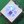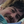0%
0%

# ALU in DCTL technology

A full ALU, capable of ADD, SUB and all logic functions, with only 12 transistors

Similar projects worth following
1.3k views
The DCTL (Direct Coupled Transistor Logic) promises quite fast logic circuits with almost no components besides the transistors themselves.
An ALU (Arithmetic Logic Unit) is a good candidate to try this technology. If I find time, a full transistor CPU might be built....

Now it is much too hot in the attic to work on my RISC Relay CPU,  thinking starts about a next project.

So I designed an ALU (wikipedia: ALU) in DCTL, that might be used in a next project. The ALU was simulated in the Falstad simulator, that has the nice property that you can actually see the current flowing during simulation.

The arithmetic and logical functions are all working correct. Optimizations for component values or speed have not been done yet.

For a discussion of DCTL, check Hackaday TTL-ers discuss DCTL and the Article of James Angell.

In a first log, I will show a full adder.

In the second log, this will be expanded to a ALU with ADD, Subtract and all 16 logic functions.

You can do the simulations yourself in the Falstad circuit simulator:  In the File menu, select "Import from text" and paste the contents of one of the files in the files section.

My 4 bit TTL ALU on one square inch or One Square Inch TTL CPU might also interest you.

Enter this text in the Falstad simulator to simulate the ALU.

plain - 7.67 kB - 08/04/2018 at 20:00

You can enter the text in the Falstad simulator to simulate the full adder.

plain - 4.78 kB - 08/04/2018 at 19:59

• 12 × NPN Transistors

• ### Or use a lot of diodes and a few transistors

For a more conventional ALU approach, using resistors in the base connections, the following adder circuit could be used as a starting point.  It could also be used for DVTL ( Diode-Vacuum-Tube-Logic).

The top part calculates the (inverted) carry. The lower part calculates the (inverted) sum, with the function   SUM =  (  ( A or B or Cin )  and /Cout  )  or  ( A and B and Cin )

The logic equivalent of this circuit is:

With a few extra parts, the carry could be forced to always be active or inactive. In that case the circuit will perform a simple AND (for carry active, /Cout=low)  or an OR (for carry not active, /Cout=high).

To connect the /Cout to Cin of the following bit, you will need an extra transistor inverter, or you could use inverted logic for every alternate bit.

To make this into a working ALU, resistor values must be calculated and some parts must be added for optimal circuit response. It is supposed that the circuits connected to the inputs can sink current but also source current (for the OR gate).

It is a simple circuit. It does not have a fast carry.

• ### Full ALU design

The full ALU design also has two parts in the schematic, the first part creates the input signals again, main difference is that now also the A signal is available in complement form:

And the following part is the actual ALU. The ALU needs both straight and inverted inputs for A and B. Since these are probably already available (because the flipflops in the CPU will have both outputs), the inverters of these signals are not included in the transistor count of the ALU.

At the lower left side, you see four switches that control the function of the ALU. The switch positions for the main functions are:

In reality, the switches should be replaced by transistors. These transistors can be in common for several bits (4 bits or perhaps even 8).

One thing must still be added to the ALU, that is a transistor that forces the input carry to zero for the logical functions. That makes it a 12-transistor design. So in this simulation, the CY-IN must be set to zero for the logic functions.

Although only seven functions are shown for the switches, the four signals can select every theoretical possible function of two binary inputs. This includes inverted versions of AND, OR and XOR, and the input B and inverted B.

The simulation can be quite surprising. Did you know that a bipolar transistor can also work backwards (current flowing between emitter and collector in opposite direction) ? This actually occurs in a certain input arrangement, but it did not have an effect on the logic output.

Not mentioned yet.... supply is 5 volts. The transistors are general NPN transistors that are available in the simulator.

This is a ripple carry adder. The carry signal has to pass T8 and T9. If this is not fast enough, I would at first check the influence of the circuit that is connected to the emitter of T8. The carry could also be made two times faster by calculating it for two bits at a time.

The Falstad simulation of the full adder has two parts. The first part creates the input signals for the adder:

There are switches to change the input signals A and B, and the Carry input signal. There are also 3 LED's to check the input values. At the left side you see the 3 created signals CY-IN, A and B, and an inverted version from B.

The second part is the actual adder:

This is actually an implementation of a classic adder design.  The following picture shows the function of the transistors:

Share

## Similar Projects

Project Owner Contributor

### LCPU - A CPU in LED-Transistor-Logic (LTL)Tim

Project Owner Contributor

### R.A.V.E.N. -- A Transistorised Brainfuck ComputerBlair Vidakovich

# Does this project spark your interest?

Become a member to follow this project and never miss any updates